• java 实现逻辑回归，附带训练集，详解回归算法-LR，二分类问题，回归问题，监督学习，因变量y和自变量x的关系 ，最小化误差平方和
• 逻辑回归-java Logistic Regression Java 类，可用于单个或多个逻辑回归分析。 通过计算所使用的每个预测变量的优势比和 logit 来估计 beta 系数。
•  逻辑回归测试类 package logisticregression; import common.ScatterPlot; public class LogisicRegressionTest { public static void main(String[] args) { double[][] sourceData = new double[][] { {...

pom.xml
<!-- 用于矩阵运算 -->
<dependency>
<groupId>org.ujmp</groupId>
<artifactId>ujmp-core</artifactId>
<version>0.3.0</version>
</dependency>
<!-- 用于显示散点图-->
<dependency>
<groupId>org.jfree</groupId>
<artifactId>jfreechart</artifactId>
<version>1.5.0</version>
</dependency>

LogisticRegression主类
package logisticregression;

import org.ujmp.core.DenseMatrix;
import org.ujmp.core.Matrix;

public class LogisticRegression {

public static double[] train(double[][] data, double[] classValues) {

if (data != null && classValues != null && data.length == classValues.length) {
Matrix matrWeights = DenseMatrix.Factory.zeros(data.length + 1, 1);
Matrix matrData = DenseMatrix.Factory.zeros(data.length, data.length + 1);
Matrix matrLable = DenseMatrix.Factory.zeros(data.length, 1);
for (int i = 0; i < data.length; i++) {
matrData.setAsDouble(1.0, i, 0);
matrLable.setAsDouble(classValues[i], i, 0);
for (int j = 0; j < data.length; j++) {
matrData.setAsDouble(data[i][j], i, j + 1);
if (i == 0) {
matrWeights.setAsDouble(1.0, j, 0);

}
}
}
matrWeights.setAsDouble(-0.5, data.length, 0);

double step = 0.01;
int maxCycle = 5000000;

for (int i = 0; i < maxCycle; i++) {
Matrix h = sigmoid(matrData.mtimes(matrWeights));
Matrix difference = matrLable.minus(h);
matrWeights = matrWeights.plus(matrData.transpose().mtimes(difference).times(step));
}

double[] rtn = new double[(int) matrWeights.getRowCount()];
for (long i = 0; i < matrWeights.getRowCount(); i++) {
rtn[(int) i] = matrWeights.getAsDouble(i, 0);
}

return rtn;

}

return null;
}

public static Matrix sigmoid(Matrix sourceMatrix) {
Matrix rtn = DenseMatrix.Factory.zeros(sourceMatrix.getRowCount(), sourceMatrix.getColumnCount());
for (int i = 0; i < sourceMatrix.getRowCount(); i++) {
for (int j = 0; j < sourceMatrix.getColumnCount(); j++) {
rtn.setAsDouble(sigmoid(sourceMatrix.getAsDouble(i, j)), i, j);
}

}

return rtn;
}

public static double sigmoid(double source) {
return 1.0 / (1 + Math.exp(-1 * source));
}

public static double getValue(double[] sourceData, double[] model) {
double logisticRegressionValue = model;
for (int i = 0; i < sourceData.length; i++) {
logisticRegressionValue = logisticRegressionValue + sourceData[i] * model[i + 1];
}
logisticRegressionValue = sigmoid(logisticRegressionValue);

return logisticRegressionValue;
}

}


逻辑回归测试类
package logisticregression;

import common.ScatterPlot;

public class LogisicRegressionTest {

public static void main(String[] args) {
double[][] sourceData = new double[][] { { -1, 1 }, { 0, 1 }, { 1, -1 }, { 1, 0 }, { 0, 0.1 }, { 0, -0.1 }, { -1, -1.1 }, { 1, 0.9 } };
double[] classValue = new double[] { 1, 1, 0, 0, 1, 0, 0, 0 };
double[] modle = LogisticRegression.train(sourceData, classValue);
double logicValue = LogisticRegression.getValue(new double[] { 0, 0 }, modle);
System.out.println("---model---");
for (int i = 0; i < modle.length; i++) {
System.out.println(modle[i]);
}
System.out.println("-----------");
System.out.println(logicValue);

double[][][] chartData = new double[][];
double[][] c0 = new double;
double[][] c1 = new double;
c1 = sourceData;
c1 = sourceData;

c1 = sourceData;
c1 = sourceData;

c0 = sourceData;
c0 = sourceData;

c0 = sourceData;
c0 = sourceData;

c1 = sourceData;
c1 = sourceData;

c0 = sourceData;
c0 = sourceData;

c0 = sourceData;
c0 = sourceData;

c0 = sourceData;
c0 = sourceData;

String[] c = new String[] { "1", "0", "L" };
double[][] c2 = new double;
int ind = 0;
for (double x = -1; x <= 1; x = x + 0.1) {
c2[ind] = x;
c2[ind] = (-modle - modle * x) / modle;
ind++;
}

chartData = c0;
chartData = c1;
chartData = c2;

ScatterPlot.showScatterPlotChart("LogisticRegression", c, chartData);

}

}



展开全文机器学习
• 使用逻辑回归对iris数据集进行分类，只选取了前2种花的部分样本。java实现。
• 逻辑回归1.1逻辑回归的概念 ...1.3逻辑回归java代码实现importjava.util.ArrayList; public class Matrix { public ArrayList<ArrayList<String>> data; public Matrix(){ data = new ArrayLis
逻辑回归
1.1逻辑回归的概念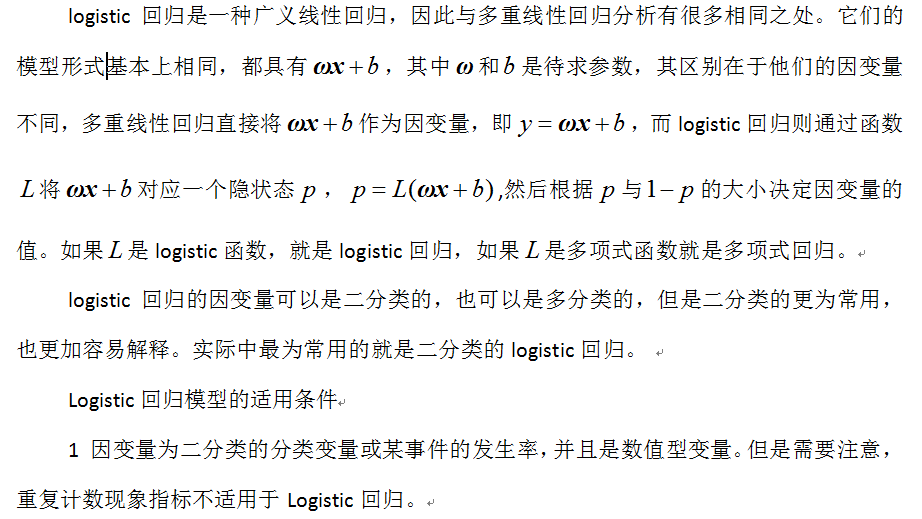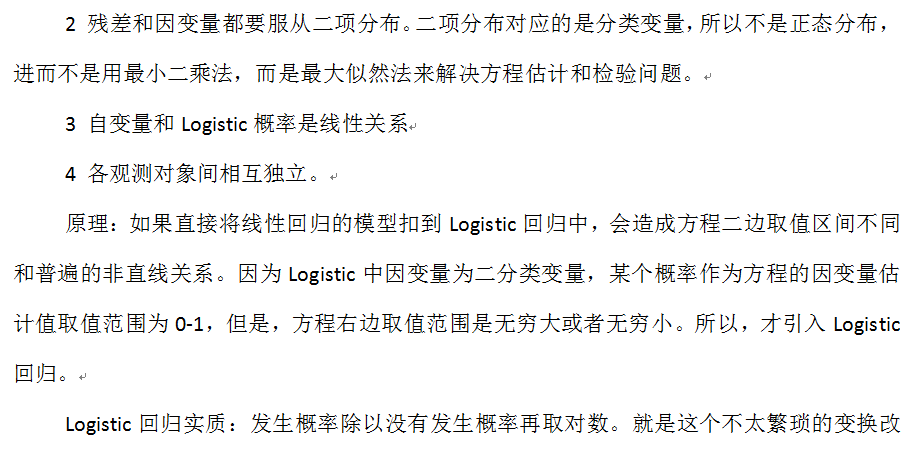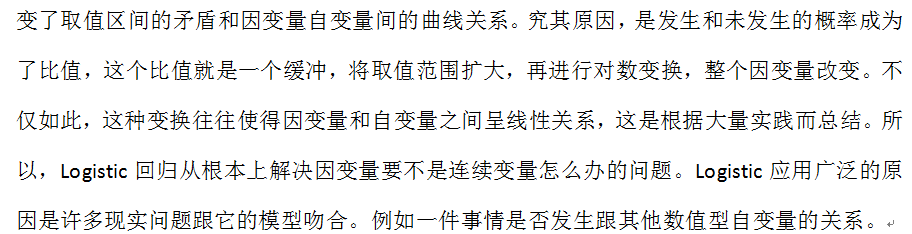1.2逻辑回归的数学表达式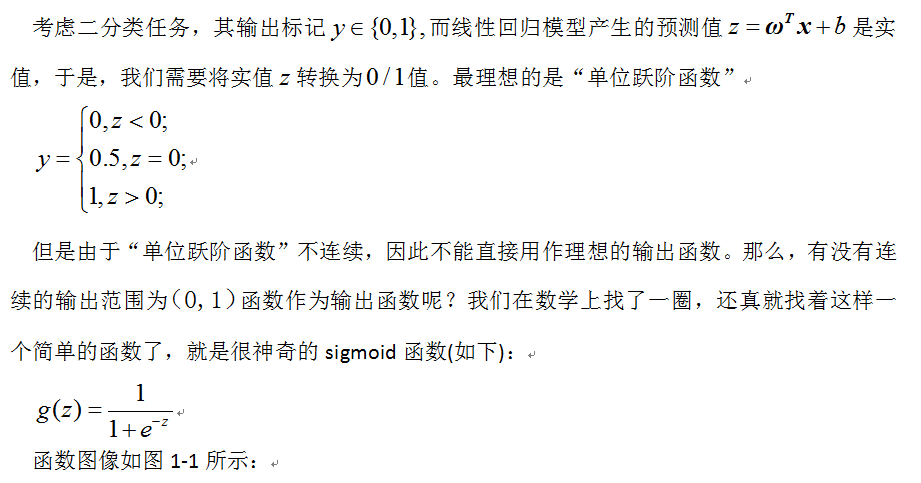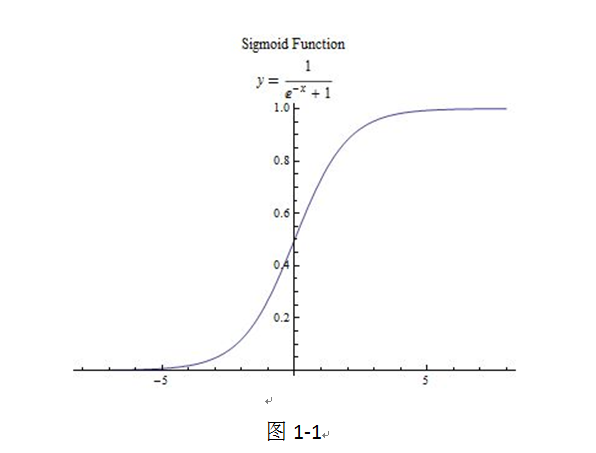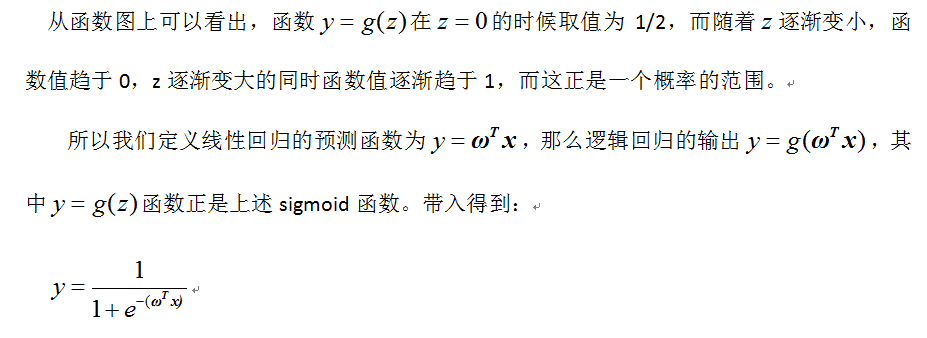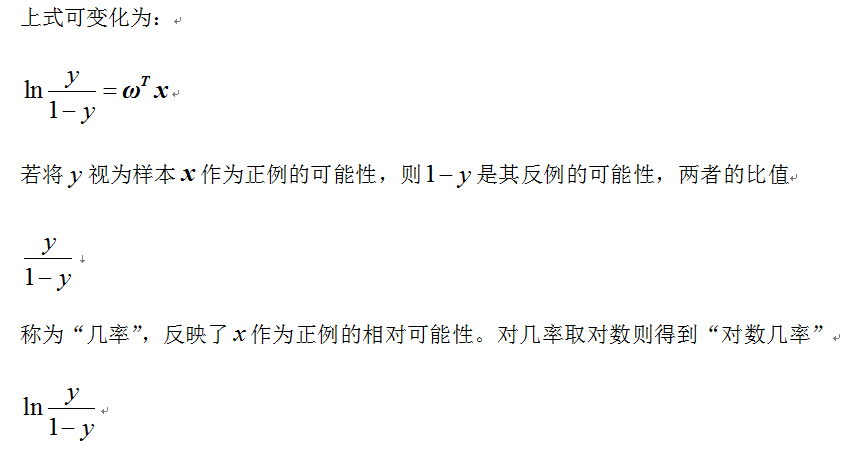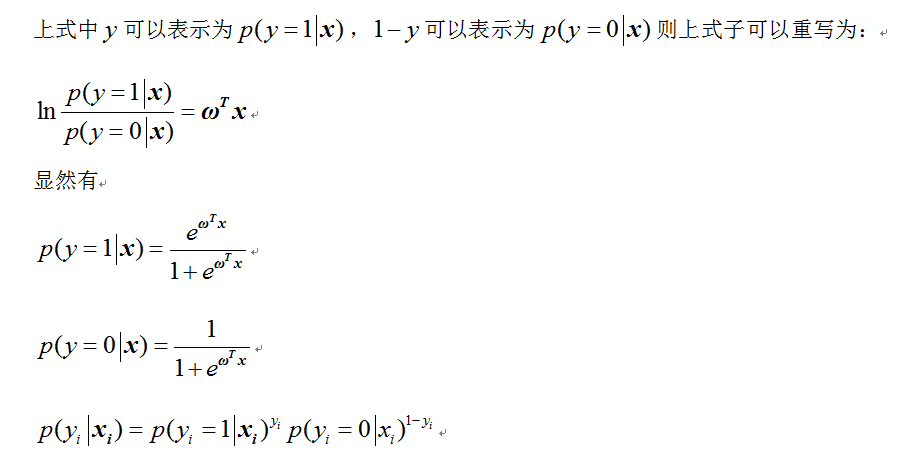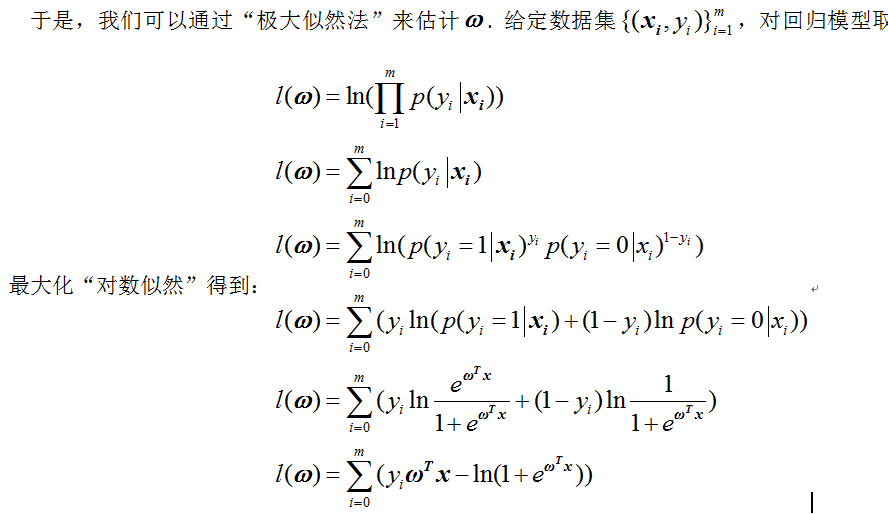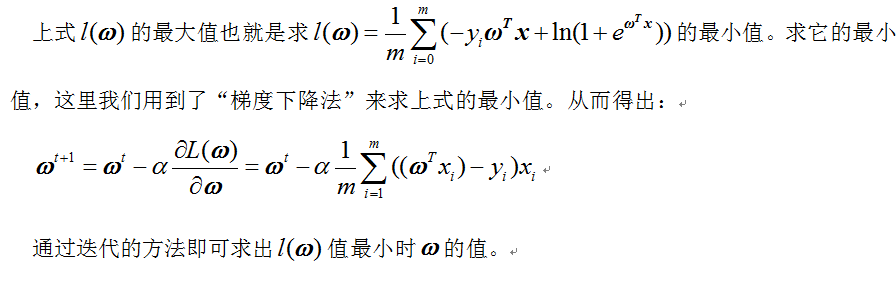1.3逻辑回归的java代码实现
importjava.util.ArrayList;
public class Matrix {
public ArrayList<ArrayList<String>> data;

public Matrix(){
data = new ArrayList<ArrayList<String>>();
}
}
import java.util.ArrayList;
public class CreateDataSet extends Matrix {
public ArrayList<String>lables;
public CreateDataSet(){
super();
lables = new ArrayList<String>();
}
public void initTest(){
}
}
import java.io.File;
import java.io.IOException;
import java.util.ArrayList;

public class Logistic {
public static void main(String[] args) {
colicTest();
}
/**
* @author haolidong
* @Description: [逻辑回归的简单测试]
*/
public static void LogisticTest() {
// TODO Auto-generated method stub
CreateDataSetdataSet = new CreateDataSet();
ArrayList<Double> weights = new ArrayList<Double>();
for (inti = 0; i< 3; i++) {
System.out.println(weights.get(i));
}
System.out.println();
}
/**
* @paraminX
* @param weights
* @return
* @author haolidong
* @Description: [sigmod分类]
*/
public static String classifyVector(ArrayList<String>inX, ArrayList<Double> weights) {
ArrayList<Double> sum = new ArrayList<>();
sum.clear();
for (inti = 0; i<inX.size(); i++) {
sum.set(0, sum.get(0) + Double.parseDouble(inX.get(i)) * weights.get(i));
}
if (sigmoid(sum).get(0) > 0.5)
return "1";
else
return "0";
}
/**
* @author haolidong
* @Description: [预测马的疝气病的死亡率]
*/
public static void colicTest() {
CreateDataSettrainingSet = new CreateDataSet();
CreateDataSettestSet = new CreateDataSet();
ArrayList<Double> weights = new ArrayList<Double>();
interrorCount = 0;
for (inti = 0; i<testSet.data.size(); i++) {
if (!classifyVector(testSet.data.get(i), weights).equals(testSet.lables.get(i))) {
errorCount++;
}
System.out.println(classifyVector(testSet.data.get(i), weights) + "," + testSet.lables.get(i));
}
System.out.println(1.0 * errorCount / testSet.data.size());
}
/**
* @paraminX
* @return
* @author haolidong
* @Description: [sigmod函数]
*/
public static ArrayList<Double> sigmoid(ArrayList<Double>inX) {
ArrayList<Double>inXExp = new ArrayList<Double>();
for (inti = 0; i<inX.size(); i++) {
}
return inXExp;
}
/**
* @paramdataSet
* @paramclassLabels
* @paramnumberIter
* @return
* @author haolidong
* @Description: [改进的随机梯度上升算法]
*/
public static ArrayList<Double> gradAscent1(Matrix dataSet, ArrayList<String>classLabels, intnumberIter) {
int m = dataSet.data.size();
int n = dataSet.data.get(0).size();
double alpha = 0.0;
intrandIndex = 0;
ArrayList<Double> weights = new ArrayList<Double>();
ArrayList<Double>weightstmp = new ArrayList<Double>();
ArrayList<Double> h = new ArrayList<Double>();
ArrayList<Integer>dataIndex = new ArrayList<Integer>();
ArrayList<Double>dataMatrixMulweights = new ArrayList<Double>();
for (inti = 0; i< n; i++) {
}
double error = 0.0;
for (int j = 0; j <numberIter; j++) {
// 产生0到99的数组
for (int p = 0; p < m; p++) {
}
// 进行每一次的训练
for (inti = 0; i< m; i++) {
alpha = 4 / (1.0 + i + j) + 0.0001;
randIndex = (int) (Math.random() * dataIndex.size());
dataIndex.remove(randIndex);
double temp = 0.0;
for (int k = 0; k < n; k++) {
temp = temp + Double.parseDouble(dataSet.data.get(randIndex).get(k)) * weights.get(k);
}
dataMatrixMulweights.set(0, temp);
h = sigmoid(dataMatrixMulweights);
error = Double.parseDouble(classLabels.get(randIndex)) - h.get(0);
double tempweight = 0.0;
for (int p = 0; p < n; p++) {
tempweight = alpha * Double.parseDouble(dataSet.data.get(randIndex).get(p)) * error;
weights.set(p, weights.get(p) + tempweight);
}
}
}
return weights;
}
File file = new File(fileName);
CreateDataSetdataSet = new CreateDataSet();
try {
String tempString = null;
// 一次读入一行，直到读入null为文件结束
// 显示行号
String[] strArr = tempString.split("\t");
ArrayList<String> as = new ArrayList<String>();
for (inti = 0; i<strArr.length - 1; i++) {
}
}
} catch (IOException e) {
e.printStackTrace();
} finally {
try {
} catch (IOException e1) {
}
}
}
return dataSet;
}
}

1.4测试结果
输入部分测试数据如下：  0 83 0 -0.7 0  0 77.39996 0 -6.3 0  1 83 0 -0.7 0  0 82.29999 0 -1.4 0  1 66.89996 0 -16.8 0  0 81 0 -2.7 0  0 87.39996 1 3.699999 0  0 82.79999 0 -0.9 0  0 84.29999 0 0.6 0  1 80.69995 0 -3 0  0 88.5 0 4.799999 0  0 80.09998 0 -3.6 0  0 83.19995 0 -0.5 0  0 88.5 0 4.799999 0  0 79.39996 0 -4.3 0  0 82.29999 0 -1.4 0  0 78.59998 0 -5.1 0  0 82.09998 0 -1.6 0  0 84.59998 0 0.9 0  0 78.19995 0 -5.5 0  0 83.69995 1 0 0  0 73.89996 0 -9.8 0  0 89.5 1 5.799999 0  0 81.29999 0 -2.4 0  0 83.09998 0 -0.6 0  第1列为lebels标签，第2、3、4、5列为属性。  输出结果为：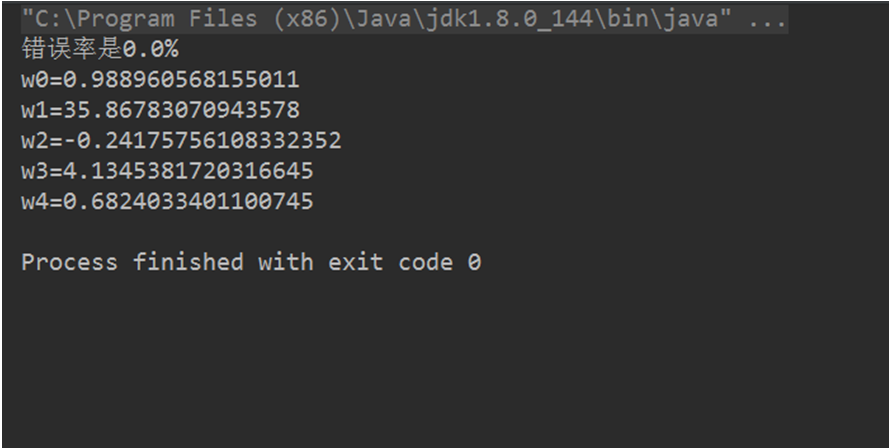回归与分类
回归问题通常是用来预测一个值，如预测房价、未来的天气情况等等，例如一个产品的实际价格为500元，通过回归分析预测值为499元，我们认为这是一个比较好的回归分析。一个比较常见的回归算法是线性回归算法（LR）。另外，回归分析用在神经网络上，其最上层是不需要加上softmax函数的，而是直接对前一层累加即可。回归是对真实值的一种逼近预测。      分类问题是用于将事物打上一个标签，通常结果为离散值。例如判断一幅图片上的动物是一只猫还是一只狗，分类通常是建立在回归之上，分类的最后一层通常要使用softmax函数进行判断其所属类别。分类并没有逼近的概念，最终正确结果只有一个，错误的就是错误的，不会有相近的概念。最常见的分类方法是逻辑回归，或者叫逻辑分类。
展开全文数学 class
• 逻辑斯蒂回归 逻辑回归 表中的内容 (Table of Content) 1. Objective 1.目的 2. Load the data 2.加载数据 3. Extract features from text 3.从文本中提取特征 4. Implementation of Logistic Regression 4.逻辑...

逻辑斯蒂回归 逻辑回归

表中的内容 (Table of Content)
1. Objective
1.目的
2.加载数据
3. Extract features from text
3.从文本中提取特征
4. Implementation of Logistic Regression
4.逻辑回归的实现
4.1 Overview 4.1概述 4.2 Sigmoid 4.2乙状结肠 4.3 Cost function 4.3成本函数 4.4 Gradient Descent 4.4梯度下降 4.5 Regularization 4.5正则化
5. Train model
5.训练模型
6. Test our logistic regression
6.测试我们的逻辑回归
7. Test with Scikit learn logistic regression
7.使用Scikit进行测试以学习逻辑回归
Let’s import all the necessary modules in Python.
让我们在Python中导入所有必需的模块。
# regular expression operationsimport re    # string operation import string  # shuffle the listfrom random import shuffle# linear algebraimport numpy as np # data processingimport pandas as pd # NLP libraryimport nltk# download twitter datasetfrom nltk.corpus import twitter_samples                          # module for stop words that come with NLTKfrom nltk.corpus import stopwords          # module for stemmingfrom nltk.stem import PorterStemmer        # module for tokenizing stringsfrom nltk.tokenize import TweetTokenizer   # scikit model selectionfrom sklearn.model_selection import train_test_split# smart progressor meterfrom tqdm import tqdm
1.目的 (1. Objective)
The goal of this kernel is to implement logistic regression from scratch for sentiment analysis using the twitter dataset. We will be mainly focusing on building blocks of logistic regression on our own. This kernel can provide an in-depth understanding of how logistic regression works internally. The notebook is converted to a medium article using the JupytertoMedium python library. The Kaggle notebook is available from here.
该内核的目标是使用Twitter数据集从头开始进行逻辑回归以进行情感分析。 我们将主要专注于我们自己的逻辑回归构建模块。 该内核可以深入了解逻辑回归在内部如何工作 。 使用JupytertoMedium python库将笔记本转换为中型文章。 Kaggle笔记本可从此处获得 。
Given a tweet, it will be classified if it has positive sentiment 👍 or negative sentiment 👎. It is very useful for beginners and others as well.
给定一条推文，如果它具有正面情感👍或负面情感👎 ，则将被分类。 对于初学者和其他人也非常有用。
# Download the twitter sample data from NLTK repositorynltk.download('twitter_samples')
The twitter_samples contains 5,000 positive tweets and 5,000 negative tweets. A total of 10,000 tweets are available. twitter_samples包含5,000条正面推文和5,000条负面推文。 共有10,000条鸣叫。 We have the same no of data samples in each class. 每个类别中的数据样本数量均相同。 It is a balanced dataset. 它是一个平衡的数据集。
# read the positive and negative tweetspos_tweets = twitter_samples.strings('positive_tweets.json')neg_tweets = twitter_samples.strings('negative_tweets.json')print(f"positive sentiment 👍 total samples {len(pos_tweets)} \nnegative sentiment 👎 total samples {len(neg_tweets)}")positive sentiment 👍 total samples 5000 negative sentiment 👎 total samples 5000# Let's have a look at the datano_of_tweets = 3print(f"Let's take a look at first {no_of_tweets} sample tweets:\n")print("Example of Positive tweets:")print('\n'.join(pos_tweets[:no_of_tweets]))print("\nExample of Negative tweets:")print('\n'.join(neg_tweets[:no_of_tweets]))Let's take a look at first 3 sample tweets:
Output:
输出：
Example of Positive tweets:#FollowFriday @France_Inte @PKuchly57 @Milipol_Paris for being top engaged members in my community this week :)@Lamb2ja Hey James! How odd :/ Please call our Contact Centre on 02392441234 and we will be able to assist you :) Many thanks!@DespiteOfficial we had a listen last night :) As You Bleed is an amazing track. When are you in Scotland?!Example of Negative tweets:hopeless for tmr :(Everything in the kids section of IKEA is so cute. Shame I'm nearly 19 in 2 months :(@Hegelbon That heart sliding into the waste basket. :(
Tweets may have URLs, numbers, and special characters. Hence, we need to preprocess the text. 推文可能包含URL，数字和特殊字符。 因此，我们需要预处理文本。
预处理文本 (Preprocess the text)
Preprocessing is one of the important steps in the pipeline. It includes cleaning and removing unnecessary data before building a machine learning model.
预处理是管道中的重要步骤之一。 它包括在建立机器学习模型之前清除和删除不必要的数据。
Preprocessing steps:
预处理步骤：
Tokenizing the string 标记字符串 Convert the tweet into lowercase and split the tweets into tokens(words) 将tweet转换为小写，并将tweet拆分为标记(单词) Removing stop words and punctuation 删除停用词和标点符号 Removing commonly used words on the twitter platform like the hashtag, retweet marks, hyperlinks, numbers, and email address 删除Twitter平台上的常用单词，例如主题标签，转发标记，超链接，数字和电子邮件地址 Stemming 抽干
It is the process of converting a word to it’s a most general form. It helps in reducing the size of our vocabulary. Example, the word engage has different stem words like, 这是将单词转换为最通用形式的过程。 它有助于减少我们的词汇量。 例如，engage这个词有不同的词干，例如 engagement 参与 engaged 投入 engaging 着迷
Let’s see how we can implement this.
让我们看看如何实现这一点。
# helper class for doing preprocessingclass Twitter_Preprocess():    def __init__(self):        # instantiate tokenizer class        self.tokenizer = TweetTokenizer(preserve_case=False, strip_handles=True,                                       reduce_len=True)        # get the english stopwords         self.stopwords_en = stopwords.words('english')         # get the english punctuation        self.punctuation_en = string.punctuation        # Instantiate stemmer object        self.stemmer = PorterStemmer()     def __remove_unwanted_characters__(self, tweet):        # remove retweet style text "RT"        tweet = re.sub(r'^RT[\s]+', '', tweet)        # remove hyperlinks        tweet = re.sub(r'https?:\/\/.*[\r\n]*', '', tweet)        # remove hashtags        tweet = re.sub(r'#', '', tweet)        #remove email address        tweet = re.sub('\S+@\S+', '', tweet)        # remove numbers        tweet = re.sub(r'\d+', '', tweet)        ## return removed text        return tweet    def __tokenize_tweet__(self, tweet):                # tokenize tweets        return self.tokenizer.tokenize(tweet)    def __remove_stopwords__(self, tweet_tokens):        # remove stopwords        tweets_clean = []        for word in tweet_tokens:            if (word not in self.stopwords_en and  # remove stopwords                word not in self.punctuation_en):  # remove punctuation                tweets_clean.append(word)        return tweets_clean    def __text_stemming__(self,tweet_tokens):        # store the stemmed word        tweets_stem = []         for word in tweet_tokens:            # stemming word            stem_word = self.stemmer.stem(word)              tweets_stem.append(stem_word)        return tweets_stem    def preprocess(self, tweets):        tweets_processed = []        for _, tweet in tqdm(enumerate(tweets)):                    # apply removing unwated characters and remove style of retweet, URL            tweet = self.__remove_unwanted_characters__(tweet)                        # apply nltk tokenizer/            tweet_tokens = self.__tokenize_tweet__(tweet)                        # apply stop words removal            tweet_clean = self.__remove_stopwords__(tweet_tokens)            # apply stemmer             tweet_stems = self.__text_stemming__(tweet_clean)            tweets_processed.extend([tweet_stems])        return tweets_processed# initilize the text preprocessor class objecttwitter_text_processor = Twitter_Preprocess()# process the positive and negative tweetsprocessed_pos_tweets = twitter_text_processor.preprocess(pos_tweets)processed_neg_tweets = twitter_text_processor.preprocess(neg_tweets)5000it [00:02, 2276.81it/s]5000it [00:02, 2409.93it/s]
Let’s take a look at what output got after preprocessing tweets. It’s good that we were able to process the tweets successfully.
让我们看一下预处理推文后得到的输出。 能够成功处理这些推文是一件好事。
pos_tweets[:no_of_tweets], processed_pos_tweets[:no_of_tweets](['#FollowFriday @France_Inte @PKuchly57 @Milipol_Paris for being top engaged members in my community this week :)',  '@Lamb2ja Hey James! How odd :/ Please call our Contact Centre on 02392441234 and we will be able to assist you :) Many thanks!',  '@DespiteOfficial we had a listen last night :) As You Bleed is an amazing track. When are you in Scotland?!'], [['followfriday', 'top', 'engag', 'member', 'commun', 'week', ':)'],  ['hey',   'jame',   'odd',   ':/',   'pleas',   'call',   'contact',   'centr',   'abl',   'assist',   ':)',   'mani',   'thank'],  ['listen', 'last', 'night', ':)', 'bleed', 'amaz', 'track', 'scotland']])
3.从文本中提取特征 (3. Extract features from text)
Given the text, It is very important to represent features (numeric values) such a way that we can feed into the model. 给定文本，以能够馈入模型的方式来表示features (numeric values)非常重要。
3.1创建单词袋(BOW)表示形式 (3.1 Create a Bag Of Words (BOW) representation)
BOW represents the word and its frequency for each class. We will create a dict for storing the frequency of positive and negative classes for each word.Let’s indicate a positive tweet is 1 and the negative tweet is 0. The dict key is a tuple containing the(word, y) pair. The word is processed word and y indicates the label of the class. The dict value represents the frequency of the word for class y.
BOW代表每个类别的单词及其出现的频率。 我们将创建一个dict来存储每个单词的positive和negative类别的频率。让我们指出一个positive推文是1和negative推文是0 。 dict键是一个包含(word, y)对的元组。 该word为已处理单词， y表示类别的标签。 dict值表示y类frequency of the word的frequency of the word 。
Example: #word bad occurs 45 time in the 0 (negative) class {(“bad”, 0) : 32}
# word bad occurs 45 time in the 0 (negative) class {("bad", 0) : 45}# BOW frequency represent the (word, y) and frequency of y classdef build_bow_dict(tweets, labels):    freq = {}    ## create zip of tweets and labels    for tweet, label in list(zip(tweets, labels)):        for word in tweet:            freq[(word, label)] = freq.get((word, label), 0) + 1    return freq# create labels of the tweets# 1 for positive labels and 0 for negative labelslabels = [1 for i in range(len(processed_pos_tweets))]labels.extend([0 for i in range(len(processed_neg_tweets))])# combine the positive and negative tweetstwitter_processed_corpus = processed_pos_tweets + processed_neg_tweets# build Bog of words frequency bow_word_frequency = build_bow_dict(twitter_processed_corpus, labels)
Now, we have various methods to represent features for our twitter corpus. Some of the basic and powerful techniques are,
CountVectorizer CountVectorizer TF-IDF feature TF-IDF功能
1. CountVectorizer (1. CountVectorizer)
The count vectorizer indicates the sparse matrix and the value can be the frequency of the word. Each column is a unique token in our corpus.
计数矢量化器指示稀疏矩阵，其值可以是单词的频率 。 每列都是我们语料库中的唯一标记。

The sparse matrix dimension would be no of unique tokens in the corpus * no of sample tweets.
稀疏矩阵维将是no of unique tokens in the corpus * no of sample tweets 。

Example: corpus = [ 'This is the first document.', 'This document is the second document.', 'And this is the third one.', 'Is this the first document?', ] and the CountVectorizer representation is
示例： corpus = [ 'This is the first document.', 'This document is the second document.', 'And this is the third one.', 'Is this the first document?', ] ，CountVectorizer表示为
[[0 1 1 1 0 0 1 0 1] [0 2 0 1 0 1 1 0 1] [1 0 0 1 1 0 1 1 1] [0 1 1 1 0 0 1 0 1]]
[[0 1 1 1 0 0 1 0 1] [0 2 0 1 0 1 1 0 1] [1 0 0 1 1 0 1 1 1] [0 1 1 1 0 0 1 0 1]]
2. TF-IDF(术语频率-反向文档频率) (2. TF-IDF (Term Frequency — Inverse Document Frequency))
TF-IDF statistical measure that evaluates how relevant a word is to a document in a collection of documents. TF-IDF is computed as follows:
TF-IDF统计量度，用于评估单词与文档集中的文档的相关性。 TF-IDF的计算如下：
TF-IDF equation

Term Frequency: term frequency tf(t,d), the simplest choice is to use the frequency of a term (word) in a document. Inverse Document Frequency: idf(t,D) a measure of how much information the word provides, i.e., if it’s common or rare across all documents. It is the logarithmic scale of the inverse fraction of the document that contains the word. The definition is as per Wiki.
术语频率：术语频率tf(t，d) ，最简单的选择是使用文档中术语(单词)的频率。 反向文档频率： idf(t，D)衡量单词提供多少信息的度量，即，该单词在所有文档中是常见还是罕见。 它是包含单词的文档反分数的对数标度 。 定义根据Wiki 。
3.2。 为我们的模型提取简单特征 (3.2. Extracting simple features for our model)
Given a list of tweets, we will be extracting two features. 给定一系列推文，我们将提取两个功能。 The first feature is the number of positive words in a tweet. 第一个功能是一条推文中肯定词的数量。 The second feature is the number of negative words in a tweet. 第二个特征是一条推文中否定词的数量。
This seems to be simple, isn’t it? Perhaps yes. We are not representing our features to the sparse matrix. Will use the simplest features for our analysis.
这似乎很简单，不是吗？ 也许是。 我们没有将特征表示为稀疏矩阵。 将使用最简单的功能进行分析。
# extract feature for tweetdef extract_features(processed_tweet, bow_word_frequency):    # feature array    features = np.zeros((1,3))    # bias term added in the 0th index    features[0,0] = 1    # iterate processed_tweet    for word in processed_tweet:        # get the positive frequency of the word        features[0,1] = bow_word_frequency.get((word, 1), 0)        # get the negative frequency of the word        features[0,2] = bow_word_frequency.get((word, 0), 0)    return features
Shuffle the corpus and will split the train and test set.
改编语料，将火车和测试仪分开。
# shuffle the positive and negative tweetsshuffle(processed_pos_tweets)shuffle(processed_neg_tweets)# create positive and negative labelspositive_tweet_label = [1 for i in processed_pos_tweets]negative_tweet_label = [0 for i in processed_neg_tweets]# create dataframetweet_df = pd.DataFrame(list(zip(twitter_processed_corpus, positive_tweet_label+negative_tweet_label)), columns=["processed_tweet", "label"])
3.3训练和测试拆分 (3.3 Train and Test split)
Let’s keep the 80% data for training and 20% data samples for testing.
让我们保留用于训练的80％数据和用于测试的20％数据样本。
# train and test splittrain_X_tweet, test_X_tweet, train_Y, test_Y = train_test_split(tweet_df["processed_tweet"], tweet_df["label"], test_size = 0.20, stratify=tweet_df["label"])print(f"train_X_tweet {train_X_tweet.shape}, test_X_tweet {test_X_tweet.shape}, train_Y {train_Y.shape}, test_Y {test_Y.shape}")train_X_tweet (8000,), test_X_tweet (2000,), train_Y (8000,), test_Y (2000,)# train X feature dimensiontrain_X = np.zeros((len(train_X_tweet), 3))for index, tweet in enumerate(train_X_tweet):    train_X[index, :] = extract_features(tweet, bow_word_frequency)# test X feature dimensiontest_X = np.zeros((len(test_X_tweet), 3))for index, tweet in enumerate(test_X_tweet):    test_X[index, :] = extract_features(tweet, bow_word_frequency)print(f"train_X {train_X.shape}, test_X {test_X.shape}")train_X (8000, 3), test_X (2000, 3)
Output:
输出：
train_X[0:5]array([[1.000e+00, 6.300e+02, 0.000e+00],       [1.000e+00, 6.930e+02, 0.000e+00],       [1.000e+00, 1.000e+00, 4.570e+03],       [1.000e+00, 1.000e+00, 4.570e+03],       [1.000e+00, 3.561e+03, 2.000e+00]])
Take a look at sample train features.
看一下样本火车功能。
The 0th index is a bias term added. 第0个索引是添加的偏差项。 1st index is representing positive word frequency 第一个索引代表正词频 2nd index is representing negative word frequency 第二个索引代表负词频率
4.逻辑回归的实现 (4. Implementation of Logistic Regression)
4.1概述 (4.1 Overview)
Now, Let’s see how logistic regression works and gets implemented.
现在，让我们看看逻辑回归是如何工作和实现的。
Most of the time, when you hear about logistic regression you may think, it is a regression problem. No, it is not, Logistic regression is a classification problem and it is a non-linear model.
大多数时候，当您听说逻辑回归时，您可能会认为这是一个回归问题。 不，不是， 逻辑回归是一个分类问题，它是一个非线性模型。
Created by Author

As shown in the above picture, there are 4 stages for most of the ML algorithms,
如上图所示，大多数ML算法有4个阶段，
Step 1. Initialize the weights
步骤1.初始化权重
Random weights initialized 随机权重已初始化
Step 2. Apply function
步骤2.应用功能
Calculate the sigmoid 计算S形
Step 3. Calculate the cost (objective of the algorithm)
步骤3.计算成本(算法的目标)
Calculate the log-loss for binary classification 计算二进制分类的对数损失
步骤4.梯度下降
Update the weights iteratively till finding the minimum cost 迭代更新权重，直到找到最低成本
Logistic regression takes a linear regression and applies a sigmoid to the output of the linear regression. So, It produces the probability of each class and it sums up to 1.
Logistic回归采用线性回归，然后将S型线应用于线性回归的输出。 因此，它产生每个类别的概率，总和为1。
Regression: Single linear regression equation as follows:
回归：单个线性回归方程如下：
Single variable Linear regression formula

Note that the theta values are weights 注意theta值是权重 x_0, x_1, x_2,… x_N is input features x_0，x_1，x_2 ...…x_N是输入要素
You may think of how complicated the equation it is. We need to multiply all the weighs with each feature at the ith position then sums up all.
您可能会想到方程式有多复杂。 我们需要将所有权重与每个特征的ith位置相乘，然后求和。

Fortunately, Linear algebra brings this equation with ease of operation. Yes, It is a matrix dot product. You can apply the dot product of features and weights to find the z.
幸运的是， 线性代数使该方程式易于操作。 是的，它是矩阵dot积。 您可以应用特征和权重的点积来找到z 。

4.2乙状结肠 (4.2 Sigmoid)
The sigmoid function is defined as: 乙状结肠功能定义为：
Sigmoid function
乙状结肠功能

It maps the input ‘z’ to a value that ranges between 0 and 1, and so it can be treated as a probability.
它将输入“ z”映射到介于0和1之间的值，因此可以将其视为概率 。
def sigmoid(z):     # calculate the sigmoid of z    h = 1 / (1+ np.exp(-z))    return h
4.3成本函数 (4.3 Cost function)
The cost function used in logistic regression is:
逻辑回归中使用的成本函数为：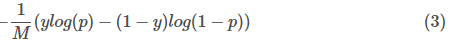This is the Log loss of binary classification. The average of the log loss across all training samples is calculated in logistic regression, the equation 3 modified for all the training samples as follows:
这是二进制分类的对数丢失。 所有训练样本的对数损失平均值通过对数回归进行计算，对所有训练样本的等式3进行了如下修改：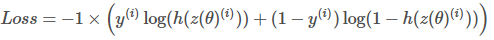m is the number of training examples m是训练示例数 y^{(i)} is the actual label of the ith training example. y ^ {(i)}是第i个训练示例的实际标签。 h(z(\theta)^{(i)}) is the model’s prediction for the ith training example. h(z(\ theta)^ {(i)})是第i个训练示例的模型预测。
The loss function for a single training example is,
单个训练示例的损失函数为
Loss function

All the h values are between 0 and 1, so the logs will be negative. That is the reason for the factor of -1 applied to the sum of the two loss terms. 所有的h   值介于0和1之间，因此对数将为负。 这就是将因子-1应用于两个损失项之和的原因。 When the model predicts 1, (h(z(θ))=1) and the label y is also 1, the loss for that training example is 0. 当模型预测1时，( h ( z ( θ ))= 1)和标签y   也为1，则该训练示例的损失为0。 Similarly, when the model predicts 0, (h(z(θ))=0) and the actual label is also 0, the loss for that training example is 0. 类似地，当模型预测为0(( h ( z ( θ ))= 0)并且实际标签也为0时，该训练示例的损失为0。 However, when the model prediction is close to 1 (h(z(θ))=0.9999) and the label is 0, the second term of the log loss becomes a large negative number, which is then multiplied by the overall factor of -1 to convert it to a positive loss value. −1×(1−0)×log(1−0.9999)≈9.2 The closer the model prediction gets to 1, the larger the loss. 但是，当模型预测接近1( h ( z ( θ ( θ ))= 0.9999)且标签为0时，对数损失的第二项变为较大的负数，然后将其乘以-的总因数1将其转换为正损耗值。 -1×(1-0)× log (1-0.9999)≈9.2模型预测值越接近1，则损失越大。
Gradient Descent is an algorithm used for updating the weights theta iteratively to minimize the objective function (cost). We need to update the weights iteratively because,
梯度下降是一种用于迭代更新权重以最小化目标函数(成本)的算法。 我们需要迭代更新权重，因为，

At initial random weights, the model doesn’t learn anything much. To improve the prediction we need to learn from the data with multiple iterations and tune the random weights accordingly.
在初始随机权重下，模型不会学到很多东西。 为了改善预测，我们需要通过多次迭代从数据中学习并相应地调整随机权重。

The gradient of the cost function J for one of the weights theta_j is:
权重theta_j之一的成本函数J的梯度为：

4.5正则化 (4.5 Regularization)
Regularization is a technique to solve the problem of overfitting in a machine learning algorithm by penalizing the cost function. There will be an additional penalty term in the cost function. There are two types of regularization techniques:
正则化是一种通过惩罚成本函数来解决机器学习算法过拟合问题的技术。 成本函数中将有一个附加的惩罚项。 有两种类型的正则化技术：
Lasso (L1-norm) Regularization 套索(L1-范数)正则化 Ridge (L2-norm) Regularization 岭(L2-范数)正则化
Lasso Regression (L1) L1-norm loss function is also known as the least absolute errors (LAE). $λ*∑ |w|$ is a regularization term. It is a product of $λ$ regularization term with an absolute sum of weights. The smaller values indicate stronger regularization.
套索回归(L1) L1范数损失函数也称为最小绝对误差(LAE)。 $λ* ∑ | w |$是正则项。 它是$λ$正则化项与绝对权重之和的乘积。 较小的值表示更强的正则化。
Ridge Regression (L2) L2-norm loss function is also known as the least squares error (LSE). $λ*∑ (w)²$ is a regularization term. It is a product of $λ$ regularization term with the squared sum of weights. The smaller values indicate stronger regularization.
岭回归(L2) L2范数损失函数也称为最小二乘误差(LSE)。 $λ* ∑(w)²$是正则项。 它是$λ$正则化项与权重平方和的乘积。 较小的值表示更强的正则化。
You could notice, that it makes a huge difference. Yes, it does well. The main difference is what type of regularization term you are adding in the cost function to minimize the error.
您可能会注意到，这有很大的不同。 是的，它做得很好。 主要区别在于您要在成本函数中添加哪种类型的正则化项以最大程度地减少误差。

L2 (Ridge) shrinks all the coefficient by the same proportions but it doesn’t eliminate any features, while L1 (Lasso) can shrink some coefficients to zero, and also performs feature selection.
L2(Ridge)将所有系数按相同比例缩小，但不会消除任何特征，而L1(Lasso)可以将某些系数缩小到零，并执行特征选择。

In the following code will add L2 regularization
在下面的代码中将添加L2正则化
# implementation of gradient descent algorithm  def gradientDescent(x, y, theta, alpha, num_iters, c):    # get the number of samples in the training    m = x.shape    for i in range(0, num_iters):        # find linear regression equation value, X and theta        z = np.dot(x, theta)        # get the sigmoid of z        h = sigmoid(z)        # calculate the cost function, log loss        #J = (-1/m) * (np.dot(y.T, np.log(h)) + np.dot((1 - y).T, np.log(1-h)))        # let's add L2 regularization        # c is L2 regularizer term        J = (-1/m) * ((np.dot(y.T, np.log(h)) + np.dot((1 - y).T, np.log(1-h))) + (c * np.sum(theta)))        # update the weights theta        theta = theta - (alpha / m) * np.dot((x.T), (h - y))    J = float(J)    return J, theta
5.训练模型 (5. Train model)
Let’s train the gradient descent function for optimizing the randomly initialized weights. The brief explanation has given in section 4.
让我们训练梯度下降函数以优化随机初始化的权重。 简要说明在第4节中给出。
# set the seed in numpynp.random.seed(1)# Apply gradient descent of logistic regression# 0.1 as added L2 regularization termJ, theta = gradientDescent(train_X, np.array(train_Y).reshape(-1,1), np.zeros((3, 1)), 1e-7, 1000, 0.1)print(f"The cost after training is {J:.8f}.")print(f"The resulting vector of weights is {[round(t, 8) for t in np.squeeze(theta)]}")The cost after training is 0.22154867.The resulting vector of weights is [2.18e-06, 0.00270863, -0.00177371]
6.测试我们的逻辑回归 (6. Test our logistic regression)
It is time to test our logistic regression function on test data that the model has not seen before.
现在该对模型从未见过的测试数据测试逻辑回归函数了。
Predict whether a tweet is positive or negative.
预测一条推文是肯定的还是负面的。
Apply the sigmoid to the logits to get the prediction (a value between 0 and 1). 将S形应用于logit以获得预测(0到1之间的值)。
Prediction for new tweets

# predict for the features from learned theata valuesdef predict_tweet(x, theta):    # make the prediction for x with learned theta values    y_pred = sigmoid(np.dot(x, theta))    return y_pred# predict for the test sample with the learned weights for logistics regressionpredicted_probs = predict_tweet(test_X, theta)# assign the probability threshold to classpredicted_labels = np.where(predicted_probs > 0.5, 1, 0)# calculate the accuracyprint(f"Own implementation of logistic regression accuracy is {len(predicted_labels[predicted_labels == np.array(test_Y).reshape(-1,1)]) / len(test_Y)*100:.2f}")Own implementation of logistic regression accuracy is 93.45
As of now, we have seen how to implement the logistic regression on our own. Got the accuracy of 94.45. Let’s see the results from the popular Machine Learning (ML) Python library.
到目前为止，我们已经看到了如何独自实现逻辑回归。 获得了94.45的准确性。 让我们看看流行的机器学习(ML)Python库的结果。
7.使用Scikit进行测试以学习逻辑回归 (7. Test with Scikit learn logistic regression)
Here, we are going to train the logistic regression from the in-build Python library to check the results.
在这里，我们将训练内置Python库中的逻辑回归以检查结果。
# scikit learn logiticsregression and accuracy score metricfrom sklearn.linear_model import LogisticRegressionfrom sklearn.metrics import accuracy_scoreclf = LogisticRegression(random_state=42, penalty='l2')clf.fit(train_X, np.array(train_Y).reshape(-1,1))y_pred = clf.predict(test_X)/opt/conda/lib/python3.7/site-packages/sklearn/utils/validation.py:73: DataConversionWarning: A column-vector y was passed when a 1d array was expected. Please change the shape of y to (n_samples, ), for example using ravel().  return f(**kwargs)print(f"Scikit learn logistic regression accuracy is {accuracy_score(test_Y , y_pred)*100:.2f}")Scikit learn logistic regression accuracy is 94.45
Great!!!. The results are pretty much close.
大！！！。 结果非常接近。
Finally, we implemented the logistic regression on our own and also tried with in-build Scikit learn logistic regression getting similar accuracy. But, this approach of feature extraction is very simple and intuitive.
最后，我们自己实现了逻辑回归，还尝试使用内置Scikit学习逻辑回归来获得相似的准确性。 但是，这种特征提取方法非常简单直观。
I am learning by doing it. Kindly leave your thoughts or any suggestions in the comments. Your feedback is highly appreciated to boost my confidence.
我正在这样做。 请在评论中留下您的想法或任何建议。 非常感谢您的反馈，以增强我的信心。

翻译自: https://towardsdatascience.com/understand-logistic-regression-from-scratch-430aedf5edb9

逻辑斯蒂回归 逻辑回归

展开全文python
• 逻辑斯蒂回归模型 java 代码 ，代码可直接运行
• 逻辑回归优点by Thalles Silva 由Thalles Silva 逻辑回归：优点 (Logistic Regression: The good parts) 您需要了解的所有信息。 (Everything you need to know about it.) Binary and Multiclass Logistic ...

逻辑回归优点
by Thalles Silva
由Thalles Silva
逻辑回归：优点 (Logistic Regression: The good parts)
您需要了解的所有信息。 (Everything you need to know about it.)
Binary and Multiclass Logistic Regression with GD and Newton’s Method
GD和牛顿法的二元和多类Logistic回归
In the last post, we tackled the problem of Machine Learning classification through the lens of dimensionality reduction. We saw how Fisher’s Linear Discriminant can project data points from higher to smaller dimensions. The projection follows two principles.
在上一篇文章中，我们通过降维的角度解决了机器学习分类的问题。 我们看到了费舍尔的线性判别式如何将数据点从较高维度投射到较小维度。 该预测遵循两个原则。
It maximizes the between-class variance.
它使类间差异最大化。

翻译自: https://www.freecodecamp.org/news/logistic-regression-the-good-parts-55efa68e11df/

逻辑回归优点

展开全文机器学习 python 人工智能
• java实现逻辑回归 基本矩阵类：Matrix package flinkjava.LR; import java.util.ArrayList; /** * 保存特征信息 * 主要保存特征矩阵 * */ public class Matrix { /** * 分为两层ArrayList * 外面代表行 * ...Flink 机器学习 性别预测 分布式计算
• 数据集X0,1,2,3,4,5,6,7,8,9,10Y0,0,0,0,0,1,1,1,1,1,1这里可以看出 当X大于4时 Y等于1逻辑回归代价函数计算公式右侧为正规化 但是这里我们并不加入正规化 因为已经足够明显了daimapackage ojama; import java.io....机器学习
• 【机器学习】Logistic Regression逻辑回归原理与java实现1、基于概率的机器学习算法2、逻辑回归算法原理2.1、分离超平面2.2、阈值函数2.3、样本概率2.4、损失函数3、基于梯度下降法的模型训练4、java实现 ...
• 作为一枚机器学习的爱好者，逻辑回归算是一个简单入门的算法，原理比较简单，但是自己手动实现逻辑回归有一些要注意的事项： 第一是步长选择的问题，根据你的数据大小来选择。 第二是自己手动可选择加不加入常数项，...
• import java.util.ArrayList; /**  *   * @author haolidong  * @Description: [该类主要用于保存特征信息]  * @parameter data: [主要保存特征矩阵]  */ public class Matrix { publ机器学习
• Java集成Weka做逻辑回归（Logistic Regression）从搜索引擎脑补可以得知，“逻辑回归”是一种分类器，通过样本集合的训练之后，可以简单做二元（或多元）分类。看了一下有用Weka做的，来来，咱也试一下。数据挖掘 weka
• 逻辑回归 数据 逻辑回归 (Logistic Regression) Logistic regression is an applied mathematics analysis methodology accustomed to predict a data price supported previous observations of a data set. ...python 机器学习 人工智能 大数据
• 本讲主要说一下逻辑回归中的几个问题和具体的参数求解方法 1. 什么是逻辑回归 2. 正则化项 3. 最小二乘法和最大似然法 4. java实现梯度下降法 实验： 样本： -0.017612 14.053064 0 -1.395634 4.662541 1 -0.752157 ...
• http://blog.csdn.net/qq_22125259/article/details/49388747  java实现的逻辑回归和其他的算法代码机器学习
• 1 逻辑回归的定位 首先，逻辑回归是一种分类（Classification）算法。比如说： 给定一封邮件，判断是不是垃圾邮件给出一个交易明细数据，判断这个交易是否是欺诈交易给出一个肿瘤检查的结果数据，判断这个肿瘤...
• ## 逻辑回归算法梳理

千次阅读 2019-04-01 21:40:48
逻辑回归算法梳理1.逻辑回归与线性回归的联系与区别2.逻辑回归的原理3.逻辑回归损失函数推导及优化4.正则化与模型评估指标5.逻辑回归的优缺点6.样本不均衡问题解决办法7.sklearn参数参考文档 1.逻辑回归与线性回归的...
• 逻辑回归二分类用到的预测函数为其中，h为预测函数(大于0.5为一类，小于等于0.5为另一类)。θ为各个特征的参数。θ=[θ1,θ2,θ3...]T损失函数J(θ)为利用梯度下降算法进行参数的更新公式如下：其中，α是学习率参数...
• 按照机器学习实战的python代码，用java重写LR的梯度上升算法： package com.log; import java.io.BufferedReader; import java.io.FileInputStream; import java.io.InputStreamReader; import java.io....
•python 深度学习
• 理论为了加深对logistic回归的理解，最好理解广义线性模型和逻辑回归的关系 斯坦福CS229机器学习课程笔记二：GLM广义线性模型与Logistic回归 从广义线性模型到逻辑回归实践源码编写Python实践之（七）逻辑回归...机器学习...

# java逻辑回归java 订阅